# AP Board 8th Class Maths Solutions Chapter 5 Comparing Quantities Using Proportion InText Questions

## AP State Syllabus 8th Class Maths Solutions 5th Lesson Comparing Quantities Using Proportion InText Questions

AP State Syllabus AP Board 8th Class Maths Solutions Chapter 5 Comparing Quantities Using Proportion InText Questions and Answers.

### 8th Class Maths 5th Lesson Comparing Quantities Using Proportion InText Questions and Answers

Do this

Question 1.
How much compound interest is earned by investing Rs. 20000 for 6 years at 5% per annum compounded annually? (Page No. 114)
P = Rs. 20,000; R = 5%; n = 6 years∴ Compound Interest = Amount – Principal = 26802 – 20,000
∴ C.I. = Rs. 6802 /-Question 2.
Find compound interest on Rs. 12600 for 2 years at 10% per annum compounded annually.    (Page No. 114)
P = Rs. 12,600; R = 10%; n = 2 years∴ Compound Interest = Amount – Principal = 15,246 – 12,600
∴ C.I. = Rs. 2646 /-

Question 3.
Find the number of conversion times the interest is compounded and rate for each.
i) A sum taken for 1$$\frac{1}{2}$$ years at 8% per annum is compounded half yearly.
ii) A sum taken for 2 years at 4% per annum is compounded half yearly.     (Page No. 115)
Compound interest will be calculated for every 6 months.
There will be 3 periods in 1$$\frac{1}{2}$$ year.
∴ n = 3
∴ Rate of interest for half yearly = $$\frac{1}{2}$$ × 8% = 4%
∴ R = 4%; n = 3
ii) C.I. should be calculated for every 6 months.
There will be 4 time periods in 2 years.
∴ n = 4
∴ Rate of interest for half yearly = $$\frac{1}{2}$$ × 4% = 2%
∴ n = 4 ; R = 2%Try These

Question 1.
Find the ratio of gear of your bicycle.       (Page No. 96)Count the number of teeth on the chain wheel and the number of teeth for the sprocket wheel.
{number of teeth on the chain wheel} : {number of teeth of sprocket wheel}
This is called gear ratio. Write how many times sprocket wheel turns for every time the chain wheel rotates once.
The ratio between the rotations of chain wheel and sprocket wheel is 4 : 1.

Question 2.
Collect newspaper cuttings related to percentages of any five different situations.  (Page No. 96)
1) Bharti to sell 5% stake for $1.2b: New Delhi, May 3: The country’s largest telecom operator Bharti Airtel said on Friday that it will sell 5 per cent stake to Doha – based Qatar Foundation Endowment (QFE) for$1.26 billion (Rs. 6,796 crores) to fund its future growth plans.
The deal will bring cash for the company at a time when its balance sheet is stretched and there is threat of Bharti Airtel having to pay hefty fees to regulatory authorities as government is re-looking at past policies.

2) Indian Firms Mop – Up Down By 36% In FY13:
New Delhi: Indian companies raised nearly Rs. 31,000 crore from the public issuance of equity and debt in 2012 – 13, a slump of 36 per cent from the preceding year.
According to latest data available with market regulator Sebi (Securities and Exchange Board of India), a total of Rs. 30,859 crore worth of fresh capital were mopped – up from equity and debt market during 2012 – 13, which was way below than Rs. 48,468 crore garnered in 2011 -12. Going by the statistics, it was mostly debt market that was leveraged to meet the funding requirements of businesses in the past fiscal as compared to capital raised through sale of shares through instruments like initial public offering (IPO) and rights issue. A total of Rs. 15,386 crore were raised from the debt market via 11 issues in 2012 – 13, much lower than Rs. 35,611 crore garnered through 20 issues in the preceding fiscal.3) IT – ITeS sector employs 2.97m people in FY13:
New Delhi: The total number of professionals working in India’s \$100 billion IT – information technology enabled services (IT – ITeS) sector grew by 7 per cent to 2.97 million in the last fiscal, Parliament was informed on Friday.
The IT – ITeS sector, which contributes about 8 per cent to the country’s economy, provided employment to 2.77 million professionals in 2011 -12 fiscal, minister of state for communications and IT Milind Deora said. “The Indian IT – ITeS industry has been progressively growing and is able to secure new projects from various foreign coun¬tries,” Mr Deora said. During the 2012 -13 fiscal, 6,40,000 professionals were employed in the domestic market.

4) For RBI, it’s not all is well yet:
Slashes repo rate by 0.25%; rules out any more cuts; raises red flag on CAD DC Correspondent Mumbai, May 3:
The RBI cut the repo rate (rate at which it lends to banks) by a quarter per cent on Friday to 7.25 per cent from 7.75 per cent, but this will not be passed on to the consumers by way of lower personal loans for housing etc., immediately according to bankers.
It also raised the growth rate from 5.2 per cent projected in January to 5.7 per cent for 2013 -14 and lowered the inflation rate to 5.5 per cent for the year.
RBI governor Dr D. Subbarao said based on the current and prospective assessment of various economic factors and the dismal 4.5 per cent lowest growth rate in the last quarter, it was decided to cut the policy rate by 25 basis points.

5) Markets sink on RBI’s Bearish outlook on rate:
DC Correspondent Mumbai, May 3:
In a highly volatile trading session, the markets retreated from their three month high led by interest rate sensitive banking, auto and real estate sector stocks after the Reserve Bank of India (RBI) cautioned that the room for further monetary policy easing is limited.
The Sensex closed 19,575.64, sliding 160.13 points or 0.81 per cent while the Nifty dropped 55.35 points or 0.92 per cent to end the week at 5,944.

Question 3.
Find the compound ratios of the following. (Page No. 99)
a) 3 : 4 and 2 : 3
b) 4 : 5 and 4 : 5
c) 5 : 7 and 2 : 9
Compound ratio of a : b and c : d is ac : bd.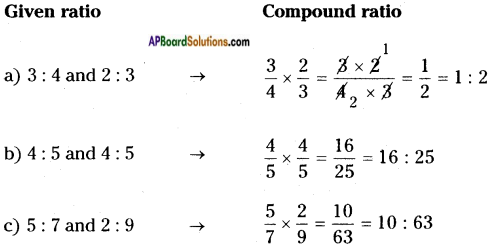Question 4.
Give examples for compound ratio from daily life.     (Page No. 99)
Examples for compound ratio from daily life:
i) To compare the ratio of tickets of 8th class students (Boys & Girls) is 3:4 and the ratio of tickets of 7th class students is 4 : 5.
ii) The comparision between two situations is 4 men can do a piece of work in 12 days, the same work 6 men can do in 8 days.
iii) Time – distance – speed.
iv) Men – days – their capacities etc.

Question 5.
Fill the selling price for each.     (Page No. 104)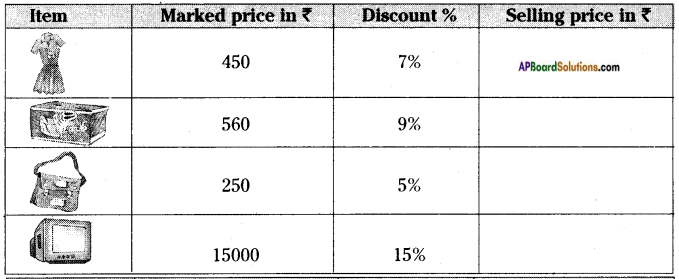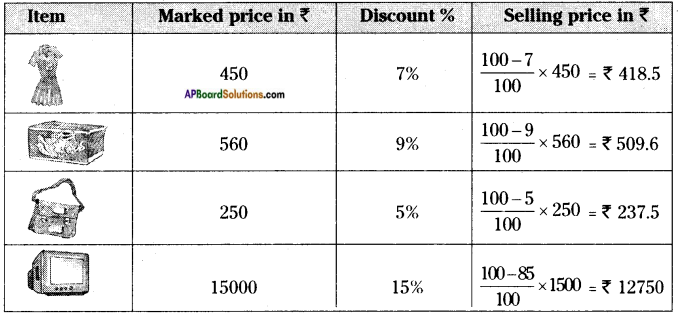Question 6.
i) Estimate 20% of Rs. 357.30 ii) Estimate 15% of Rs. 375.50      (Page No. 105)ii) 15% of 375.50 = $$\frac{15}{100}$$ × 375.50 = 15 × 3.7550 = Rs. 56.325

Question 7.
Complete the table.     (Page No. 105)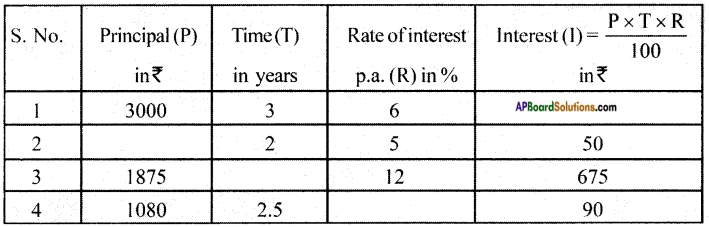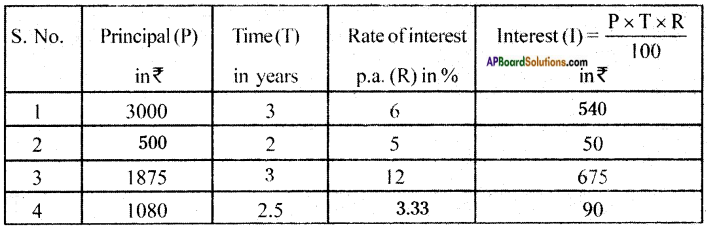Think, discuss and write

Question 1.
Two times a number is 100% increase in the number. If we take half the number what would be the decrease in percent?    (Page No. 101)
Increase percent of 2 times of a number = $$\frac{(2-1)}{1}$$ × 100 = 1 × 100 = 100%
Half of the number = 1 – $$\frac{1}{2}$$ = $$\frac{1}{2}$$
Decrease in percent = $$\frac{\frac{1}{2}}{1}$$ × 100 = $$\frac{1}{2}$$ × 100 = 50%Question 2.
By what percent is Rs. 2000 less than Rs. 2400? Is it the same as the percent by which Rs. 2400 is more than Rs. 2000? (Page No. 101)
Decrease in percent of Rs. 2000 less than Rs. 2400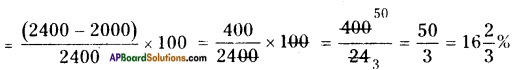Increase in percent of Rs. 2400 more than Rs. 2000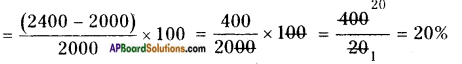Question 3.
Preethi went to a shop to buy a dress. Its marked price is Rs. 2500. Shop owner gave 5% discount on it. On further insistence, he gave 3% more discount. What will be the final discount she obtained? Will it be equal to a single discount of 8%? Think, discuss with your friends and write it in your notebook. (Page No. 105)
Marked price of a dress selected by Preethi = Rs. 2500
After allowing 5% of discount then S.P = M.P. – Discount%
= 2500 – $$\frac{5}{100}$$ × 2500 = 2500 – 125 = Rs. 2375
Again 3% discount is allowed on Rs. 2375 then
S.P = 2375 – 3% of 2375
= 2375 – $$\frac{3}{100}$$ × 2375 = 2375 – 71.25 = Rs. 2303.75
If 8% discount is allowed then S.P =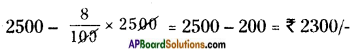The S.P’s of both cases are not equal.
Discount on 5% + Discount on 3% = 125 + 71.25 = Rs. 196.25
Discount on 8% = Rs. 200
∴ Discounts are not equal which are obtained by Preethi.

Question 4.
What happens if cost price = selling price. Do we get any such situations in our daily life?
It is easy to find profit % or loss% in the above situations. But it will be more meaningful if we express them in percentages. Profit % is an example of increase percent of cost price and loss % is an example of decrease percent of cost price. (Page No. 106)
If selling price is equal to cost price then either profit or loss will not be occurred.
In our daily life S.P. will not be equal to C.P. Then profit or loss will be occurred.
∴ Profit % = $$\frac{\text { Profit }}{\text { C.P. }}$$ × 100;
Loss % = $$\frac{\text { Loss }}{\text { C.P. }}$$ × 100.Question 5.
A shop keeper sold two TV sets at Rs. 9,900 each. He sold one at a profit of 10% and the other at a loss of 10%. Oh the whole whether he gets profit or loss? If so what is its percentage? (Page No. 108)
S.P of each T.V = Rs. 9,900
S.P of both T.Vs = 2 × 9,900 = Rs. 19,800
10% profit is allowed on first then C.P. =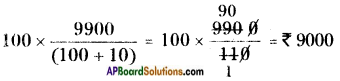10% loss is allowed on second then C.P.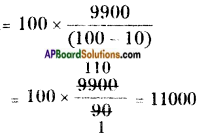C.P. of both T.V.’s = 9000 + 11000 = Rs. 20,000
Here C.P > S.P then loss will be occurred.
∴ Loss = C.P – S.P = 20000 – 19,800 = 200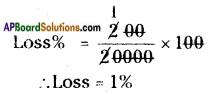Question 6.
What will happen if interest is compounded quarterly? How many conversion periods will be there? What about the quarter year rate – how much will it be of the annual rate? Discuss with your friends. (Page No. 115)
Rate of Interest (R) = $$\frac{R}{4}$$ [∵ $$\frac{12}{3}$$ = 4]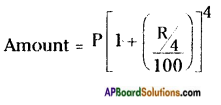A = P$$\left[1+\frac{R}{400}\right]^{4}$$